Start typing, then use the up and down arrows to select an option from the list.Jules Bruno
430views
3
Hey, guys, In this brand new video, we're gonna take a look at the equilibrium. Constant. Now we're going to say that the variable we usedto illustrate our equilibrium. Constant is the variable K. We're gonna say K is a number equal to the ratio of products to react. It's at a given temperature. Why do I say at a given temperature? Because we're gonna say that temperature directly effects are equilibrium, constant K increasing the temperature will increase our K value. Decreasing our temperature will decrease r k value. Now we're gonna say it's a ratio of products to react in. So we're gonna say here that K equals products, overreact INTs. Now we're gonna say that K is important because it it's magnitude tells us how far to the left or to the right are. Chemical reaction is at a given temperature. So we're gonna say if K is greater than one than products, our favorite overreact. It's and think about it. This makes sense because we said K equals products. Overreactions. So let's say our products are 10 on our reactant are one k equals 10 definitely greater than one. We're gonna say when products are favorite over our reactant. That means we're making more products. How do we make more products by going in the forward direction so the four direction would be favored now in the opposite way if K is less than one? So let's say we have. In this case, products are one, but our reactions air 10 we have a K less than one. So in this case, if K is less than one than react INTs our favorite over products, which means our reaction is heading in the reverse direction so the reverse direction would be favored. So I just remember these two differences when K is greater than one when K is less than one Now, what if we say Kay was equal toe one? We know that this is products, overreact INTs. So that means that our products and reactant would have to be equal. Let's say they're both 10. 10/10 is one. So when K equals one, we're gonna say both are reacting in our product. Amounts are equal to one another. Now we're gonna say the equilibrium constant K takes into account all the states of matter except to it doesn't look at solids, and it doesn't look at liquids. It ignores those two states for matter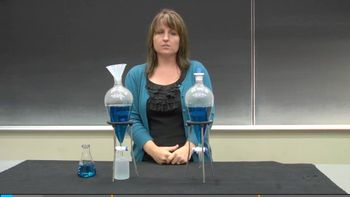3:49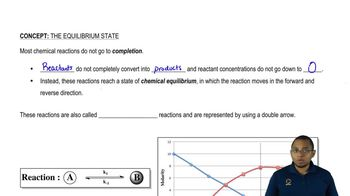03:42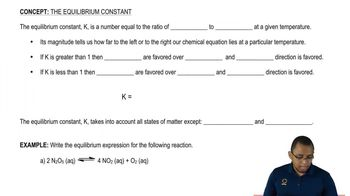02:50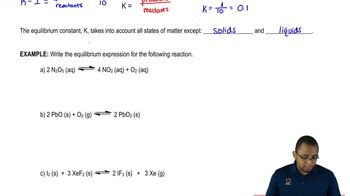03:43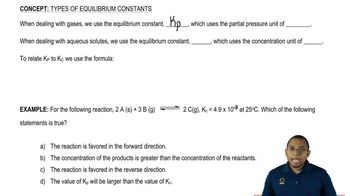03:01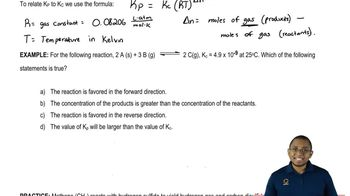03:35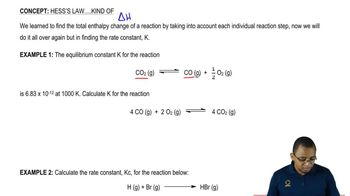03:58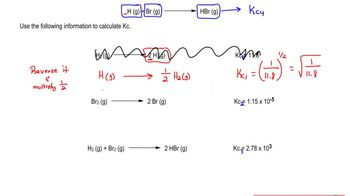06:46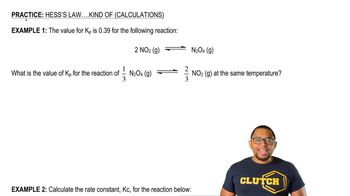03:39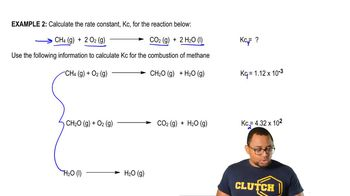03:28